# Unsupervised Training

## Producer Field Guide

HGD_Product
Producer Field Guide
HGD_Portfolio_Suite
Producer

Unsupervised training requires only minimal initial input from you. However, you have the task of interpreting the classes that are created by the unsupervised training algorithm.

Unsupervised training is also called clustering, because it is based on the natural groupings of pixels in image data when they are plotted in feature space. According to the specified parameters, these groups can later be merged, disregarded, otherwise manipulated, or used as the basis of a signature.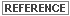See Feature Space in Dimensionality of Data for more information.

Clusters

Clusters are defined with a clustering algorithm, which often uses all or many of the pixels in the input data file for its analysis. The clustering algorithm has no regard for the contiguity of the pixels that define each cluster.

• Iterative Self-Organizing Data Analysis Technique (ISODATA) (Tou and Gonzalez, 1974) clustering method uses spectral distance as in the sequential method, but iteratively classifies the pixels, redefines the criteria for each class, and classifies again, so that the spectral distance patterns in the data gradually emerge.
• RGB clustering method is more specialized than the ISODATA method. It applies to three-band, 8-bit data. RGB clustering plots pixels in three-dimensional feature space, and divides that space into sections that are used to define clusters.

Each of these methods is explained below, along with its advantages and disadvantages.Some statistics terms used in this section are explained in Math Topics.

ISODATA Clustering

ISODATA is iterative in that it repeatedly performs an entire classification (outputting a thematic raster layer) and recalculates statistics. Self-Organizing refers to the way in which it locates clusters with minimum user input.

The ISODATA method uses minimum spectral distance to assign a cluster for each candidate pixel. The process begins with a specified number of arbitrary cluster means or the means of existing signatures, and then it processes repetitively, so that those means shift to the means of the clusters in the data.

Because the ISODATA method is iterative, it is not biased to the top of the data file, as are the one-pass clustering algorithms.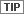Use the Unsupervised Classification dialog in Signature Editor to perform ISODATA clustering.

ISODATA Clustering Parameters

To perform ISODATA clustering, you specify:

• N - maximum number of clusters to be considered. Since each cluster is the basis for a class, this number becomes the maximum number of classes to be formed. The ISODATA process begins by determining N arbitrary cluster means. Some clusters with too few pixels can be eliminated, leaving less than N clusters.
• T - a convergence threshold, which is the maximum percentage of pixels whose class values are allowed to be unchanged between iterations.
• M - maximum number of iterations to be performed.

Initial Cluster Means

On the first iteration of the ISODATA algorithm, the means of N clusters can be arbitrarily determined. After each iteration, a new mean for each cluster is calculated, based on the actual spectral locations of the pixels in the cluster, instead of the initial arbitrary calculation. Then, these new means are used for defining clusters in the next iteration. The process continues until there is little change between iterations (Swain, 1973).

The initial cluster means are distributed in feature space along a vector that runs between the point at spectral coordinates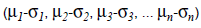and the coordinates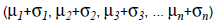Such a vector in two dimensions is illustrated in the figure below. The initial cluster means are evenly distributed between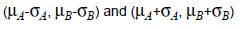The figure below shows five arbitrary cluster means in two-dimensional spectral space.

ISODATA Arbitrary Clusters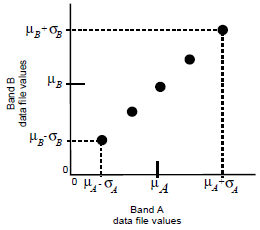Pixel Analysis

Pixels are analyzed beginning with the upper left corner of the image and going left to right, block by block.

The spectral distance between the candidate pixel and each cluster mean is calculated. The pixel is assigned to the cluster whose mean is the closest. The ISODATA function creates an output image file with a thematic raster layer and/or a signature file (.sig) as a result of the clustering. At the end of each iteration, an image file exists that shows the assignments of the pixels to the clusters.

Considering the regular, arbitrary assignment of the initial cluster means, the first iteration of the ISODATA algorithm always gives results similar to those in the figure below.

ISODATA First Pass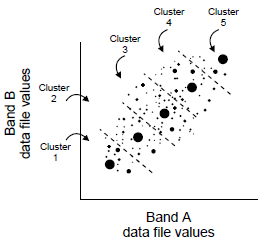For the second iteration, the means of all clusters are recalculated, causing them to shift in feature space. The entire process is repeated—each candidate pixel is compared to the new cluster means and assigned to the closest cluster mean.

ISODATA Second Pass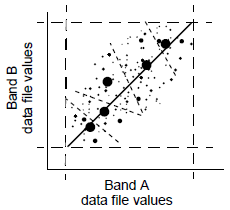Percentage Unchanged

After each iteration, the normalized percentage of pixels whose assignments are unchanged since the last iteration is displayed in the dialog. When this number reaches T (the convergence threshold), the program terminates.

It is possible for the percentage of unchanged pixels to never converge or reach T (the convergence threshold). Therefore, it may be beneficial to monitor the percentage, or specify a reasonable maximum number of iterations, M, so that the program does not run indefinitely.

 Advantages Disadvantages Because it is iterative, clustering is not geographically biased to the top or bottom pixels of the data file. Clustering process is time-consuming, because it can repeat many times. This algorithm is highly successful at finding the spectral clusters that are inherent in the data. It does not matter where the initial arbitrary cluster means are located, as long as enough iterations are allowed. Does not account for pixel spatial homogeneity. A preliminary thematic raster layer is created, which gives results similar to using a Minimum Distance classifier (as explained below) on the signatures that are created. This thematic raster layer can be used for analyzing and manipulating the signatures before actual classification takes place.

Principal Component Method

Whereas clustering creates signatures depending on pixels’ spectral reflectance by adding pixels together, the principal component method actually subtracts pixels. Principal Components Analysis (PCA) is a method of data compression. With it, you can eliminate data that is redundant by compacting it into fewer bands.

The resulting bands are noncorrelated and independent. You may find these bands more interpretable than the source data. PCA can be performed on up to 256 bands with ERDAS IMAGINE. As a type of spectral enhancement, you are required to specify the number of components you want output from the original data.

Recommended Decision Rule

Although the ISODATA algorithm is the most similar to Minimum Distance decision rule, the signatures can produce good results with any type of classification. Therefore, no particular decision rule is recommended over others.

In most cases, the signatures created by ISODATA are merged, deleted, or appended to other signature sets. The image file created by ISODATA is the same as the image file that is created by a Minimum Distance classification, except for the nonconvergent pixels (100-T% of the pixels).Use the Merge and Delete options in Signature Editor to manipulate signatures.Use the Unsupervised Classification dialog in Signature Editor to perform ISODATA clustering, generate signatures, and classify the resulting signatures.

RGB Clustering

RGB Clustering and Advanced RGB Clustering functions create a thematic raster layer. However, no signature file is created and no other classification decision rule is used. In practice, RGB Clustering differs greatly from the other clustering methods, but it does employ a clustering algorithm.

RGB clustering is a simple classification and data compression technique for three bands of data. It is a fast and simple algorithm that quickly compresses a three-band image into a single band pseudocolor image, without necessarily classifying any particular features.

The algorithm plots all pixels in 3-dimensional feature space and then partitions this space into clusters on a grid. In the more simplistic version of this function, each of these clusters becomes a class in the output thematic raster layer.

The advanced version requires that you set a minimum threshold on the clusters so that only clusters at least as large as the threshold become output classes. This allows for more color variation in the output file. Pixels that do not fall into any of the remaining clusters are assigned to the cluster with the smallest city-block distance from the pixel. In this case, the city-block distance is calculated as the sum of the distances in the red, green, and blue directions in 3-dimensional space.

Along each axis of the three-dimensional scatterplot, each input histogram is scaled so that the partitions divide the histograms between specified limits—either a specified number of standard deviations above and below the mean, or between the minimum and maximum data values for each band.

The default number of divisions per band is listed below:

• Red is divided into 7 sections (32 for advanced version)
• Green is divided into 6 sections (32 for advanced version)
• Blue is divided into 6 sections (32 for advanced version)

RGB Clustering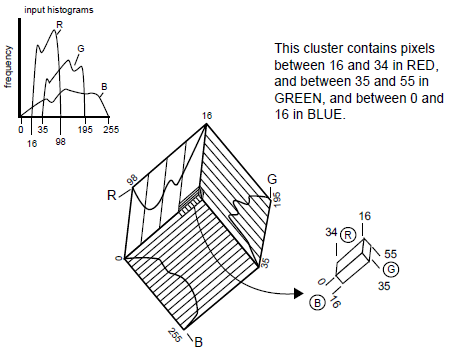Partitioning Parameters

It is necessary to specify the number of R, G, and B sections in each dimension of the 3-dimensional scatterplot. The number of sections should vary according to the histograms of each band. Broad histograms should be divided into more sections, and narrow histograms should be divided into fewer sections, as shown in the figure above.

It is possible to interactively change these parameters in RGB Clustering function. The number of classes is calculated based on the current parameters, and it displays on the command screen.

 Advantages Disadvantages Fastest classification method. It is designed to provide a fast, simple classification for applications that do not require specific classes. Exactly three bands must be input, which is not suitable for all applications. Not biased to the top or bottom of the data file. The order in which the pixels are examined does not influence the outcome. Does not always create thematic classes that can be analyzed for informational purposes. (Advanced version only) A highly interactive function, allowing an iterative adjustment of the parameters until the number of clusters and the thresholds are satisfactory for analysis.

Tips

Some starting values that usually produce good results with the simple RGB clustering are:

R = 7

G = 6

B = 6

which results in 7 × 6 × 6 = 252 classes.

To decrease the number of output colors/classes or to darken the output, decrease these values.

For the Advanced RGB clustering function, start with higher values for R, G, and B. Adjust by raising the threshold parameter or decreasing the R, G, and B parameter values until the desired number of output classes is obtained.